# RD Sharma Class 10 Solutions Chapter 13 Areas Related to Circles Ex 13.3

## RD Sharma Class 10 Solutions Chapter 13 Areas Related to Circles Ex 13.3

These Solutions are part of RD Sharma Class 10 Solutions. Here we have given RD Sharma Class 10 Solutions Chapter 13 Areas Related to Circles Ex 13.3

Other Exercises

Question 1.
AB is a chord of a circle with centre O and radius 4 cm. AB is of length 4 cm and divides the circle into two segments. Find the area of the minor segment.
Solution:
Radius of the circle (r) = 4 cm
Length of the chord AB = 4 cm
∴  In ΔOAB
OA = OB = AB    (each = 4 cm)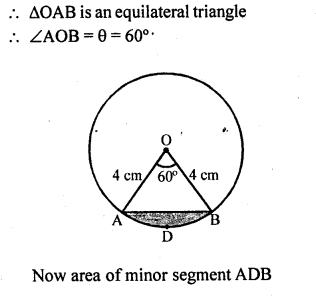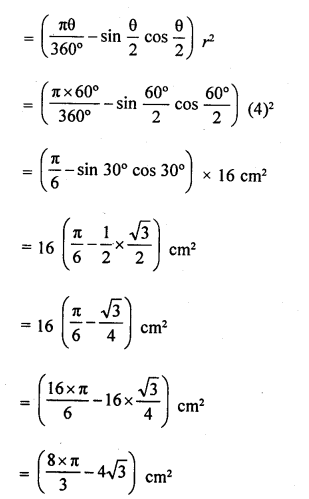Question 2.
A chord PQ of length 12 cm subtends an angle of 120° at the centre of a circle. Find the area of the minor segment cut off by the chord PQ.
Solution:
Length of chord PQ = 12 cm
Angle at the centre (θ) = 120°
∵  Draw OD ⊥ DQ
which bisects PQ at D and also bisects ∠POQ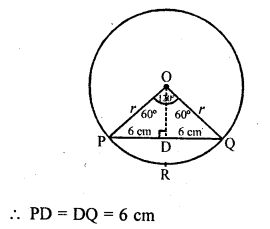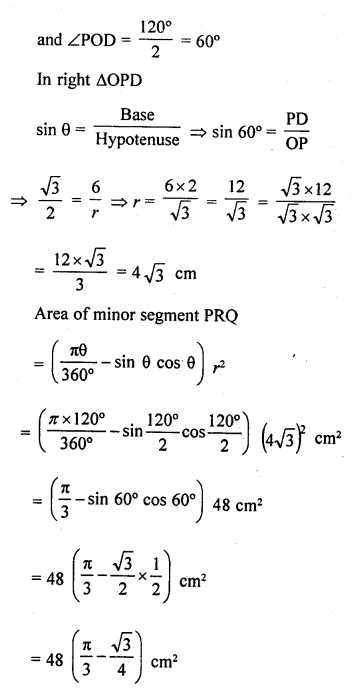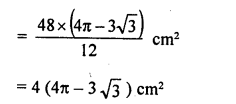Question 3.
A chord of a circle of radius 14 cm makes a right angle at the centre. Find the areas of the minor and major segments of the circle.
Solution:
Radius of the circle (r) = 14 cm
Angle at the centre (θ) = 90°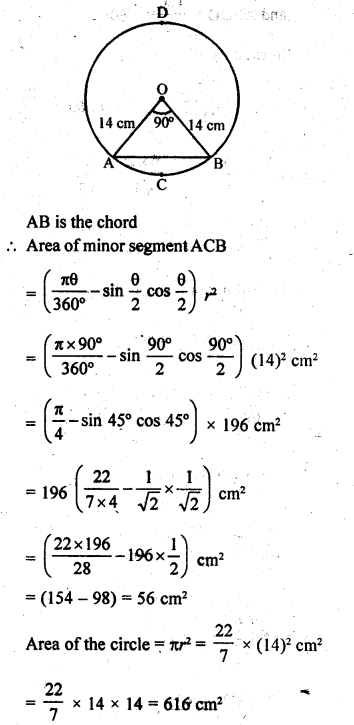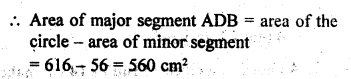Question 4.
A ehord 10 cm long is drawn in a circle whose radius is 5$$\sqrt { 2 }$$
cm. Find area of both the segments. (Take π = 3.14).

Solution:
Radius of the circle (r) = 5$$\sqrt { 2 }$$  cm
And length of chord AB = 10 cm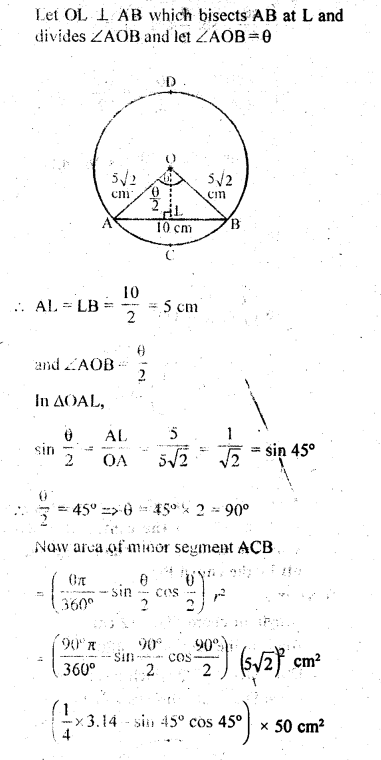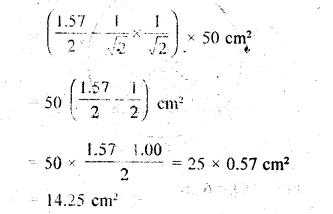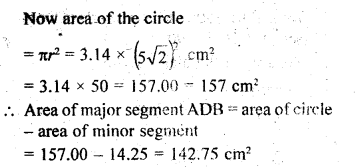Question 5.
A chord AB of a circle, of radius 14 cm makes an angle of 60° at the centre of the circle. Find the area of the minor segment of the circle. (Use π = 22/7)
Solution:
Radius of the circle (r) – 14 cm
Angle at the centre subtended in the fnui
AB = 60°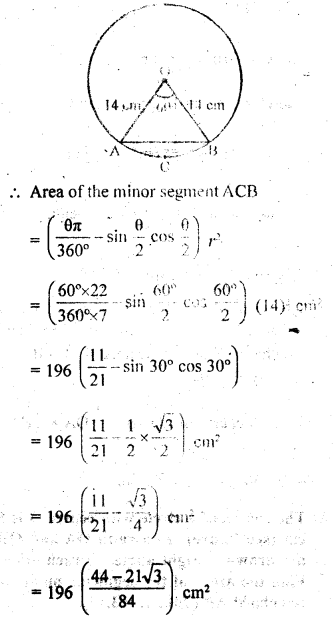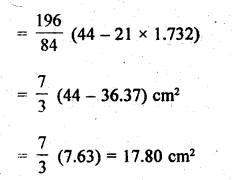Question 6.
Find the area of the minor segment of a circle of radius 14 cm, when the angle of the corresponding sector is 60°. [NCERT Exemplar]
Solution:
Given that, radius of circle (r) = 14 cm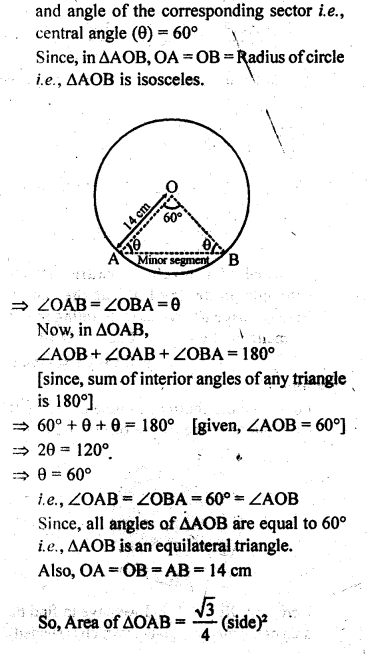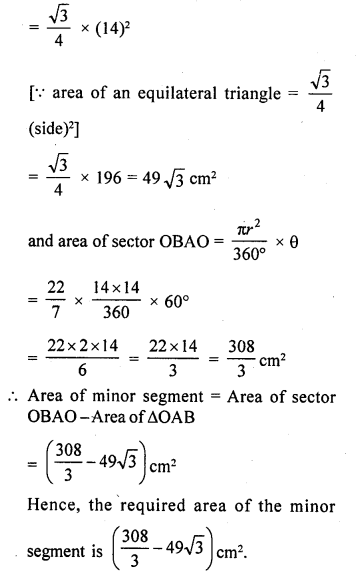Question 7.
A chord of a circle of radius 20 cm subtends an angle of 90° at the centre. Find the area of the corresponding major segment of the circle. (Use π = 3.14) [NCERT Exemplar]
Solution:
Let AB be the chord of a circle of radius 10 cm,
with O as the centre of the circle.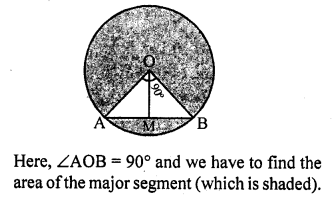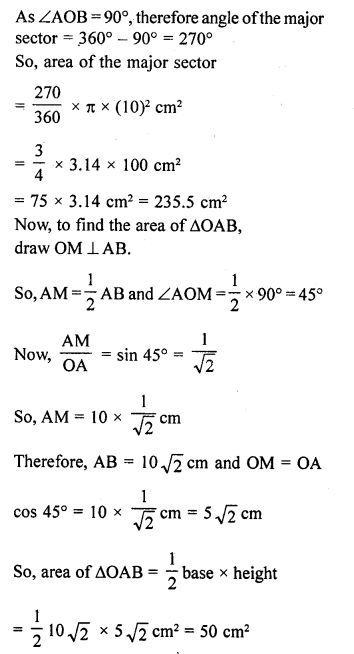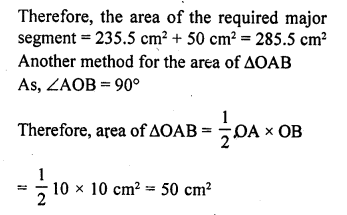Question 8.
The radius of a circle with centre O is 5 cm (see figure). Two radii OA and OB are drawn at right angles to each other. Find the areas of the segments made by the chord AB (Take π = 3.14).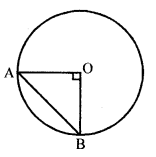Solution:
Radius of the circle (r) = 5 cm
∵   OA and OB are at right angle
∴ ∠AOB = 90°
∵  Chord AB makes two segments which are minor segment and major segment Now area of minor segment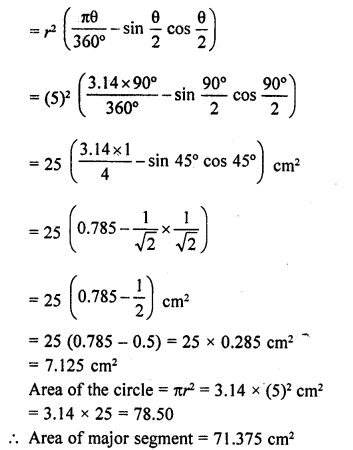Question 9.
AB is the diameter of a circle, centre O. C is a point on the circumference such that ∠COB = 0. The area of the minor segment cut off by AC is equal to twice the area of the sector BOC. Prove that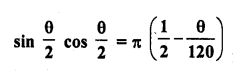Solution: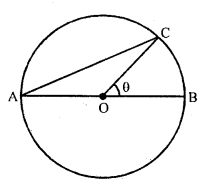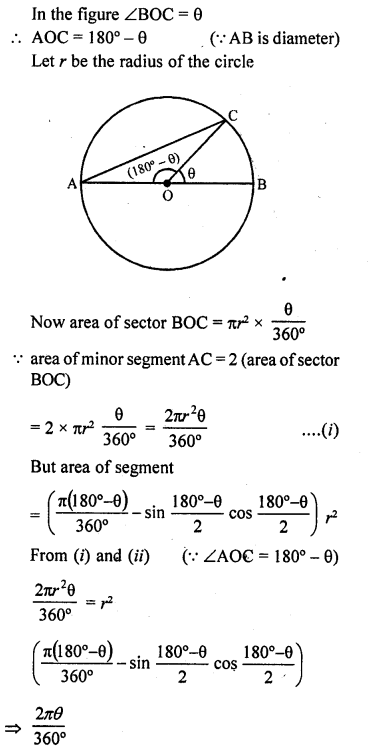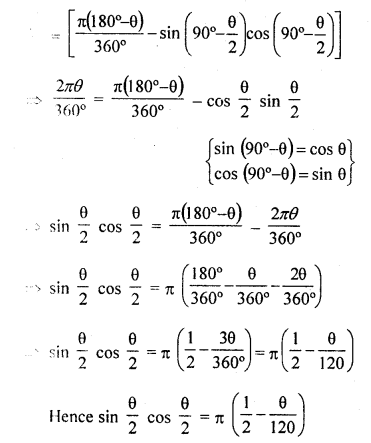Question 10.
A chord of a circle subtends an angle of 0 at the centre of the circle. The area of the minor segment cut off by the chord is one eighth of the area of the circle. Prove that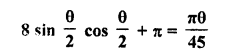Solution:
Let chord AB subtends angle 0 at the centre
of a circle with radius r
Now area of the circle = nr1
and area of the minor segment ACB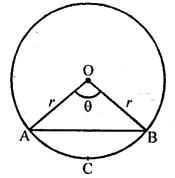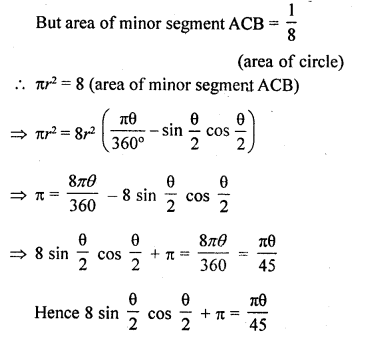Hope given RD Sharma Class 10 Solutions Chapter 13 Areas Related to Circles Ex 13.3 are helpful to complete your math homework.

If you have any doubts, please comment below. Learn Insta try to provide online math tutoring for you.